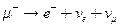Chapter 33, Problem 29PE

Chapter
Section
Textbook Problem

Is the decaypossible considering the appropriate conservation laws? State why or why not.

To determine

Whether the decay μe+νe+νμ is possible as per the conservation laws.

Explanation

Given info:

Given reaction is μe+νe+νμ

Calculation:

We can verify whether the decay μe+νe+νμ is possible by checking the following conservation laws.

Decay:μe+νe+νμ

Charge: 1  1  00conserved

B:  0  0  00

Still sussing out bartleby?

Check out a sample textbook solution.

See a sample solution

The Solution to Your Study Problems

Bartleby provides explanations to thousands of textbook problems written by our experts, many with advanced degrees!

Get Started